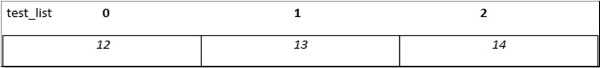# Dart Programming - Lists

#### Dart tutorial for Beginners

44 Lectures 4.5 hours

#### Flutter Tutorial for Beginners with Dart

34 Lectures 4 hours

#### Dart Masterclass Programming: iOS/Android Bible

69 Lectures 4 hours

A very commonly used collection in programming is an array. Dart represents arrays in the form of List objects. A List is simply an ordered group of objects. The dart:core library provides the List class that enables creation and manipulation of lists.

The logical representation of a list in Dart is given below −• test_list − is the identifier that references the collection.

• The list contains in it the values 12, 13, and 14. The memory blocks holding these values are known as elements.

• Each element in the List is identified by a unique number called the index. The index starts from zero and extends up to n-1 where n is the total number of elements in the List. The index is also referred to as the subscript.

Lists can be classified as −

• Fixed Length List
• Growable List

Let us now discuss these two types of lists in detail.

## Fixed Length List

A fixed length list’s length cannot change at runtime. The syntax for creating a fixed length list is as given below −

Step 1 − Declaring a list

The syntax for declaring a fixed length list is given below −

```var list_name = new List(initial_size)
```

The above syntax creates a list of the specified size. The list cannot grow or shrink at runtime. Any attempt to resize the list will result in an exception.

Step 2 − Initializing a list

The syntax for initializing a list is as given below −

```lst_name[index] = value;
```

### Example

Live Demo
```void main() {
var lst = new List(3);
lst = 12;
lst = 13;
lst = 11;
print(lst);
}
```

It will produce the following output

```[12, 13, 11]
```

## Growable List

A growable list’s length can change at run-time. The syntax for declaring and initializing a growable list is as given below −

Step 1 − Declaring a List

```var list_name = [val1,val2,val3]
--- creates a list containing the specified values
OR
var list_name = new List()
--- creates a list of size zero
```

Step 2 − Initializing a List

The index / subscript is used to reference the element that should be populated with a value. The syntax for initializing a list is as given below −

```list_name[index] = value;
```

### Example

The following example shows how to create a list of 3 elements.

Live Demo
```void main() {
var num_list = [1,2,3];
print(num_list);
}
```

It will produce the following output

```[1, 2, 3]
```

### Example

The following example creates a zero-length list using the empty List() constructor. The add() function in the List class is used to dynamically add elements to the list.

Live Demo
```void main() {
var lst = new List();
print(lst);
}
```

It will produce the following output

```[12, 13]
```

## List Properties

The following table lists some commonly used properties of the List class in the dart:core library.

Sr.No Methods & Description
1 first

Returns the first element in the list.

2 isEmpty

Returns true if the collection has no elements.

3 isNotEmpty

Returns true if the collection has at least one element.

4 length

Returns the size of the list.

5 last

Returns the last element in the list.

6 reversed

Returns an iterable object containing the lists values in the reverse order.

7 Single

Checks if the list has only one element and returns it.